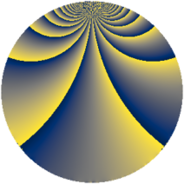# Properties

 Label 168.2.vLevel $168$ Weight $2$ Character orbit 168.v Rep. character $\chi_{168}(11,\cdot)$ Character field $\Q(\zeta_{6})$ Dimension $56$ Newform subspaces $1$ Sturm bound $64$ Trace bound $0$

# Related objects

## Defining parameters

 Level: $$N$$ $$=$$ $$168 = 2^{3} \cdot 3 \cdot 7$$ Weight: $$k$$ $$=$$ $$2$$ Character orbit: $$[\chi]$$ $$=$$ 168.v (of order $$6$$ and degree $$2$$) Character conductor: $$\operatorname{cond}(\chi)$$ $$=$$ $$168$$ Character field: $$\Q(\zeta_{6})$$ Newform subspaces: $$1$$ Sturm bound: $$64$$ Trace bound: $$0$$

## Dimensions

The following table gives the dimensions of various subspaces of $$M_{2}(168, [\chi])$$.

Total New Old
Modular forms 72 72 0
Cusp forms 56 56 0
Eisenstein series 16 16 0

## Trace form

 $$56 q - 2 q^{3} - 2 q^{4} - 8 q^{6} - 2 q^{9} + O(q^{10})$$ $$56 q - 2 q^{3} - 2 q^{4} - 8 q^{6} - 2 q^{9} + 6 q^{10} + 10 q^{12} - 10 q^{16} - 10 q^{18} - 4 q^{19} - 20 q^{22} - 8 q^{24} - 16 q^{25} - 8 q^{27} - 22 q^{28} - 12 q^{30} - 14 q^{33} - 56 q^{34} + 4 q^{36} + 14 q^{40} - 8 q^{42} - 16 q^{43} - 12 q^{46} + 64 q^{48} - 16 q^{49} - 34 q^{51} - 8 q^{52} + 32 q^{54} + 4 q^{57} - 18 q^{58} + 40 q^{60} + 4 q^{64} - 20 q^{66} - 36 q^{67} - 42 q^{70} + 22 q^{72} + 4 q^{73} + 104 q^{76} + 28 q^{78} - 10 q^{81} + 52 q^{82} + 4 q^{84} + 46 q^{88} + 140 q^{90} + 72 q^{91} - 46 q^{96} - 32 q^{97} - 44 q^{99} + O(q^{100})$$

## Decomposition of $$S_{2}^{\mathrm{new}}(168, [\chi])$$ into newform subspaces

Label Dim $A$ Field CM Traces $q$-expansion
$a_{2}$ $a_{3}$ $a_{5}$ $a_{7}$
168.2.v.a $56$ $1.341$ None $$0$$ $$-2$$ $$0$$ $$0$$